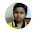## Sunday, July 29, 2018

It is very interesting logical image question for teens. In this logical puzzle there is some logical equation statements which has some hidden logical pattern. This hidden logical pattern relates the numbers given on both the sides of the equation. Can you find this hidden pattern and solve the last equation?Can you solve logical Image Question?

Answer of this "Logical Image Question for Teens", can be viewed by clicking on button. Please do give your best try before looking at the answer.

1.1.No. Your answer is different from our solution. It will be great if you can write down your logic to solve this puzzle?

2.1.3.Equation process pleass

1.Answer to this logical question is explained in this post. Please let me know if you have any doubts?

4.5.6.8=88 .... 8x8=64 + (8+8) 16 + 8 = 88 ......

A comment doesn't cost a thing. Please drop a comment below to boost the author's morale.